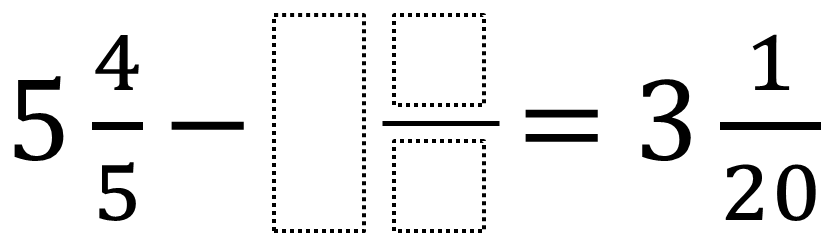Home > Grade 5 > Subtracting Mixed Numbers

# Subtracting Mixed Numbers

Directions: Create three different mixed numbers that will make the equation true by using the whole numbers from 1 to 9, no more than one time each. You may reuse the same numbers for each of the three mixed numbers.### Hint

How can I use the first answer I find to find additional answers?